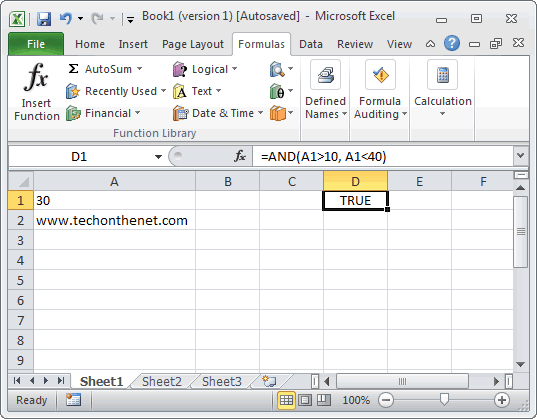# Learn To Use The AND Function in Excel WS

## Recent Posts

In this Excel tutorial, we at DexLab Analytics will dig a little deeper with our Excel knowledge and explain how to use the Excel AND function with proper examples, and syntax use.

### What is the AND function?

The MS Excel AND function works to return TRUE if all the conditions are true. And it returns FALSE if any of the conditions are false. The AND function is an internal function in Excel that is categorized as a Logical Function. It can be utilised as a Work Sheet (WS) function in Excel. Moreover, as a worksheet function, the AND function may as well be included as a part of a formula in a normal excel cell of a worksheet.

For the VBA version of this function, stay tuned to our regular blogs as we will be discussing the VBA version of the AND function as well, the syntax for that is very different.

Seeking Excel dashboards training Pune? DexLab Analytics is now in Pune.### Syntax:

For using the AND function in MS Excel the syntax goes something like this:

`AND( condition1, [condition2], ... )`

### Arguments or Parameters:

The first condition:

The first condition, is to determine whether it is TRUE or FALSE

The second condition:

This second condition is optional and includes additional conditions to test whether are TRUE or FALSE. There may be a total of up to 30 conditions in total.

### What type of function is AND used here as?

This is a WS (Work Sheet) function.

### Use case example as a Work Sheet function:Here we take a look at some Excel AND functions as an example and look at how to use the AND function as a worksheet function in Microsoft Excel.

Depending upon the Excel spreadsheet above, when we put the AND function it will return the following:

```=AND(A1>10, A1<40)
Result: TRUE

=AND(A1=30, A2="www.checkyourmath.com")
Result: FALSE

=AND(A1>=5, A1<=30, A2="www.techonthenet.com")
Result: TRUE```

### Common queries about AND function in WS Excel:

Q1: I need to translate some Quattro Pro functions into MS Excel. Is there any way the #AND# function in Qpro may be placed in the midst of a nest and return with a number. Like for instance, @if (…A1>B1#and#A1<B3, 7, 0)

What this formula means is after use of some functions if A1 is greater than that of B1 and A1 is less than B3, then it will return with 7 or otherwise, 0.

This may be done in Excel by combining the AND function with the IF function in this following way:

`=IF(AND(A1>B1,A1<B3)=TRUE,7,0)`

Q2: When in Ms Excel, i often try to use the IF function to return 25 if in case Cell A1 is > 100 and the Cell B1 < 200. Or else it should return with zero.

One can make use of the AND function to perform the AND condition in the IF function as mentioned below:

`=IF(AND(A1>100,B1<200),25,0)`

In this given example, the formula will return 25 if the condition mentioned above of which, is if cell A1 > 100 and Cell B1 < 200, or else it will return with zero.

For Advanced excel training in Delhi check out our premiere advanced Excel VBA institute – DexLab Analytics.

This post originally appeared onwww.techonthenet.com/excel/formulas/and.php

#### Interested in a career in Data Analyst?### Call us to know more### Gurgaon+91 931 572 5902